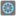• # Even the last - failed on extra 11

## Question related to missionEven the Last

def checkio(array):

```leng = len(array)
result = 0
if leng == 0:
return (0)
else:
for item in array:
if array.index(item) % 2 == 0 and not item == 0:
result +=  item
return (result * (array[leng-1]))
```

This is my code. The weird thing is that it works for every test apart from the last one (Extra 11) where it gives me quite a lot of negative numbers and positive numbers. My answer is 500 away from what it is supposed to be. I don't see why my code should fail on this one but work on all the others. Even the ones with negative numbers. If someone could help me I'd be very grateful.4